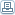# What is Gravity?

 By: Burt JordaanAll of us know the mysterious force called gravity, holding us firmly onto Earth's surface. However, the question 'what is gravity' is not easy to answer at all. The reason is that we don't really understand what this force actually is (if it is indeed a force). What follows may give you a different way of thinking about gravity.Let's see if the three 'gravity giants', Kepler, Newton and Einstein did answer the question 'what is gravity?'Kepler's Gravity (1605)Johannes Kepler published the 'laws' of planetary motion in 1605, after studying the precise measurement of the orbits of the planets by Tycho Brahe. He found that these observations followed three relatively simple mathematical laws, i.e.1. The orbit of every planet is an ellipse with the Sun at one of the two focus points.2. A line joining a planet and the Sun sweeps out equal areas during equal intervals of time.3. The squares of the orbital periods of planets are directly proportional to the cubes of the major axis (half the "length" of the ellipse) of the orbits.The physical explanation of this behavior of the planets came almost a century later when Sir Isaac Newton was able to deduce Kepler's laws from his laws of motion and his law of universal gravitation.Newton's Gravity (1687)Newton, reportedly while observing an apple falling from a tree, got an inspiration that allowed him to work out how the force of gravity can be described mathematically. In his 'Principia' of 1687, Isaac Newton included his famous three laws of motion and also the law of 'universal gravitation'. They can be briefly stated as:1. An object in motion will remain in motion unless acted upon by a net force.2. Force equals mass multiplied by acceleration.3. To every action there is an equal and opposite reaction.4. The force of gravity between two point masses is proportional to the product of the two masses and inversely proportional to the square of the distance between them.Double one of the two masses and the force of gravity between them will also double. Double the distance between the masses and the force of gravity will be four times weaker.It is now known that Newton's universal gravitation does not fully describe the effects of gravity when the gravitational field is very strong, or when objects move at very high speed in the field. This is where Einstein's general theory of relativity rules.Einstein's Gravity (1916)Einstein reportedly got the inspiration for his imaginative leap in the understanding of gravity by contemplating a man falling off a building. Such a falling man would not experience any force while he is falling, at least not before hitting the ground and suffering severe forces.In his monumental 1916 work 'The Foundation of the General Theory of Relativity', Albert Einstein unified his own special theory of relativity and Newton's law of universal gravitation with his crucial insight - the effects of gravity may not be a force, but can be described by the curvature of space and time.It is reasonably easy to accept that space can be curved – after all, we all know that a disk has a curved edge, but how can time be 'curved'? The secret lurks in the way that space and time is combined into space-time. Normally, a space-time diagram is drawn with a straight horizontal spatial axis and a straight vertical time axis. Just bend the two straight axes a little and we have curved space-time.It's not quite that simple, but the effects of gravity can be viewed as no more than an arrow of spatial movement and an arrow of time movement that are both slightly bent. The bending is accomplished by the energy locked up in every massive object. It has been stated that 'mass tells space-time how to curve and curved space-time tells mass how to move'.SummaryHave we got an answer to the question 'what is gravity?' Not quite. At the most fundamental level, no one really knows. We can say that it is an effect that keeps planets and moons in their orbits. We can say that it is a force that makes one mass attract another mass or we can say that it is what controls the movement of matter through curved space and time. Lately, we can also say that it is (perhaps) a manifestation of 'quantum gravity' - but that's another story…